×

Search anything:

# Biconnected graph

#### Algorithms Graph AlgorithmsGet this book -> Problems on Array: For Interviews and Competitive Programming

A biconnected graph is a graph that is connected and has no articulation points.

Contents

1. Introduction
2. Algorithm to check if graph is biconnected.
3. Applications of biconnected graph.

Connected
A graph is said to be connected if there is a path between every pair of vertices of the graph.

The following graph is connected since one vertex can be visited from any other vertex and at least one path exists between every pair of vertices.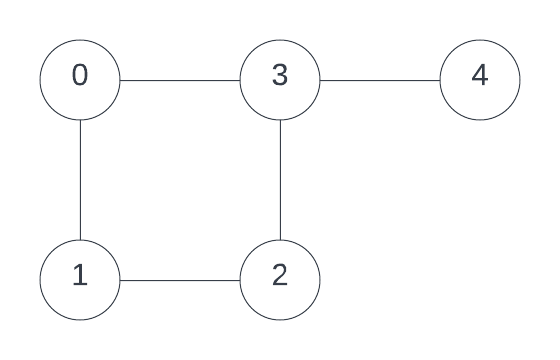The following graph is disconnected as it has two independent components which are disconnected, that is there exists at least one pair of vertices that don't have a path between them.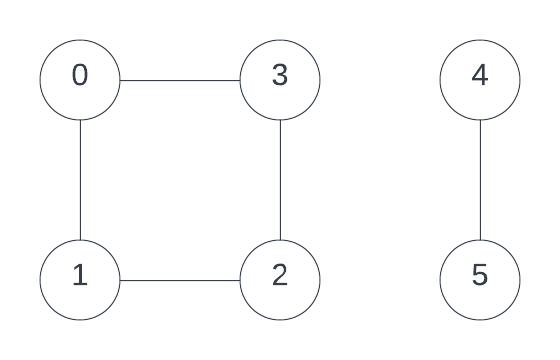Articulation points or cut vertices.
An articulation point is a vertex in the graph if the removal of it and the corresponding edges cause the graph to be disconnected. Consider the graph G in the following figure.
Vertices = { 0,1,2,3,4 } Edges = { {0,1}, {1,3}, {2,3}, {2,4}, {3,4} }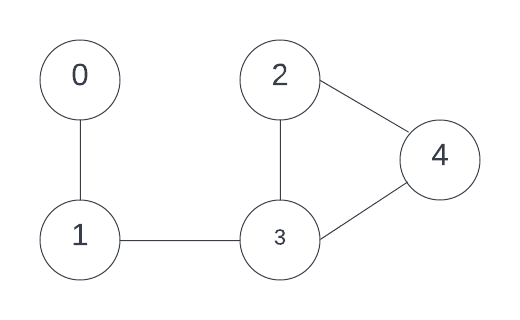• Vertex 1 is an articulation point since the removal of it causes the graph to be disconnected. The associated edges also removed are { {0,1}, {1,3} }.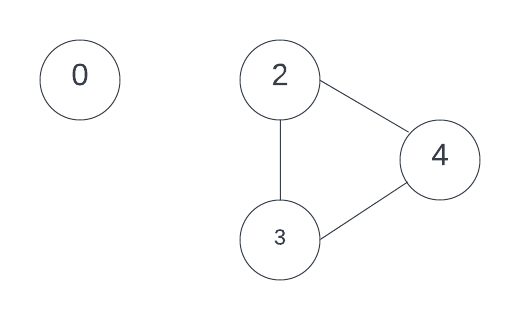• Vertex 3 is also an articulation point since the removal of it causes the graph to be disconnected. The associated edges also removed are { {1,3}, {2,3}, {3,4} }.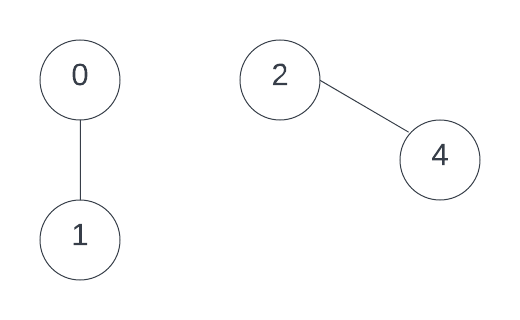Biconnected graph

The following graph is a biconnected graph. The graph is connected and doesn't have any articulation points.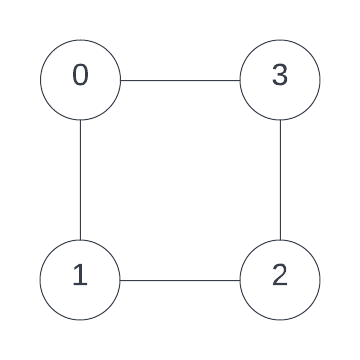The following graph is not a biconnected graph as it has an articulation point which is vertex "2".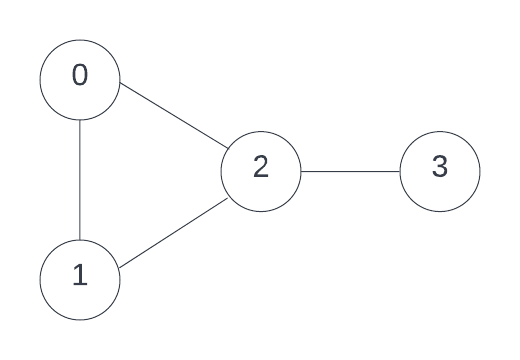## Algorithm to check if graph is biconnected

Tarjan's algorithm

1. Assume that Graph G is an undirected connected graph.
2. We find the biconnected components of the graph G using depth first spanning tree which is found using depth first search on a start vertex of G.
3. To maintain the order in which vertices are visited or to store the time of discovering each vertex, we use a vector called dfn here which stands for depth first number.
4. In the depth first spanning tree where u and v are vertices and u is an ancestor of v, then dfn(u) < dfn(v).
5. A non-tree edge {u, v} is a back-edge if and only if either u is an ancestor of v or vice versa. All non-tree edges are back-edges.
6. To check if a vertex is an articulation point:
• Given vertex is root: the root of a depth first spanning tree is an articulation point if and only if has at least two child nodes.
• Given vertex is not root: A vertex v can be an articulation point if it has a child u and the subtree rooted at u doesn't have a back-edge to any of the ancestors of v.
7. This means for a non-root node v, if at least one of its descendants u exists such that an ancestor of v cannot be reached from u using just one single back edge through u or through descendants of u.
8. To store the information about the node with the lowest discovery time accessible from a given node using atmost one back-edge, we use a vector named low.
9. For every edge, we check for the lowest low[] value possible for the node
low[node] = min ( low[node], low [child_node])
10. If the edge is a back-edge then
low[node] = min (low[node],discover_time[child_node])
11. Now for a given node v we need to compare the low[] value of a child node u with the depth first number of v. If if the low[] value of a child node u is is greater than or equal to the discovery time or depth first number of v then v is an articulation point.
low[u] >= dfn[v] or low[child_node_of_vertex] >= dfn[vertex] then v is an articulation point.
12. We need to check if all the vertices reachable from a given vertex of graph are reachable from every other vertex in the graph on removal of the given vertex to confirm connectivity also.

Program

``````// Part of iq.opengenus.org
#include<bits/stdc++.h>

using namespace std;

vector<int>G;

void dfsMod(int u, vector<int>& dfn, vector<int>& low, vector<int>& parent, vector<int>& cut_vertices, vector<int>& visited, int& time)
{
int children_u = 0;
visited[u] = 1;
dfn[u] = low[u] = time;
time++;

for(int child : G[u])
{
if(dfn[child] == -1)
{
parent[child] = u;
children_u++;

dfsMod(child, dfn, low, parent, cut_vertices, visited, time);
low[u] = min(low[u], low[child]);

if (parent[u] == -1 && children_u > 1)
{
cut_vertices[u] = 1;
}

else if (parent[u] != -1 && low[child] >= dfn[u])
{
cut_vertices[u] = 1;
}
}

else if(parent[u] != child){
low[u] = min(low[u], dfn[child]);
}
}
}

void cut_vertices(int v, int e)
{
vector<int>parent(v + 1, -1);
vector<int>dfn(v + 1, -1);
vector<int>low(v + 1, -1);

vector<int>cut_vertices(v + 1, 0);
vector<int>visited(v+1, 0);
int time = 1;
int ap = 0;
int conn = 1;

for (int i = 0; i <= v; i++)
{
if (dfn[i] == -1)
{
dfsMod(i, dfn, low, parent, cut_vertices, visited, time);
time = 1;
}
}
for(int i =0;i <v;i++)
{
if(visited[i] == 0){
cout << "The graph is not connected and hence not biconnected";
conn = 0;
}

if (cut_vertices[i] == 1)
{
ap++;
}
}
if(ap !=0){
cout << "The graph is not biconnected and has articulation points." <<endl;
cout << "Articulation points :";

for(int i=1;i<=v; i++)
{
if (cut_vertices[i] == 1)
{
cout << i << " ";

}
}
}

if(ap==0 and conn == 1)
{
cout << "The graph is biconnected.";
}
}

int main()
{
int v, e;
v = 5, e = 5;

G.push_back(1);
G.push_back(0);
G.push_back(3);
G.push_back(3);
G.push_back(4);
G.push_back(2);
G.push_back(2);
G.push_back(4);
G.push_back(2);
G.push_back(3);

cut_vertices(v, e);
}
``````

Output
The graph is not biconnected and has articulation points.
Articulation points: 1 3

Time complexity to check if a graph is biconnected is O(V + E) since depth first search traversal is used in the graph. Here V is the number of nodes and E is the number of edges in the graph.

Graph G is the graph in the following figure:The spanning tree formed from the dfs traversal would be: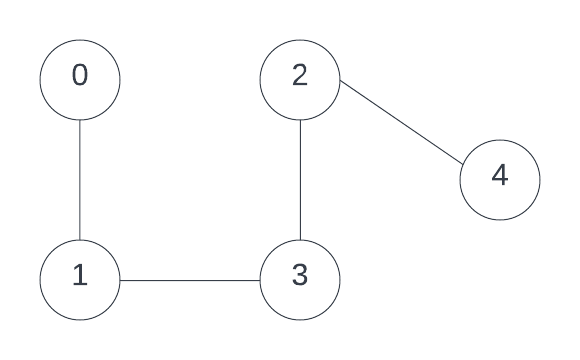Initially the low values are initialized to the node's depth first number.
For every edge, the values of low of each node are revised to the lowest depth first number value of the node reachable from the given node without visiting the nodes already visited. The red lines represent the paths already visited.

for non-back edge: low[node] = min ( low[node], low [child_node])
for back edge: low[node] = min (low[node],discover_time[child_node])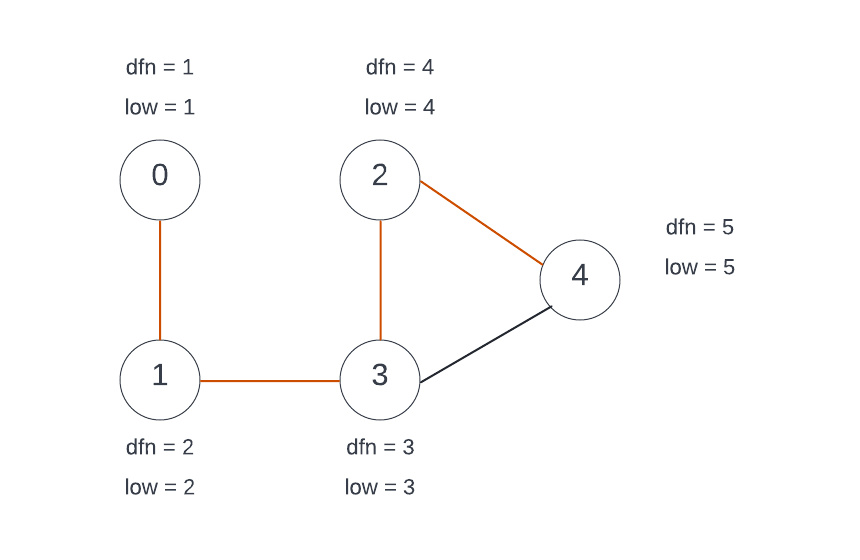Now we can see that for the node "4", the lowest depth first number reachable is of node "3", hence low = 3. The green path represents the path to the node with lowest depth first number.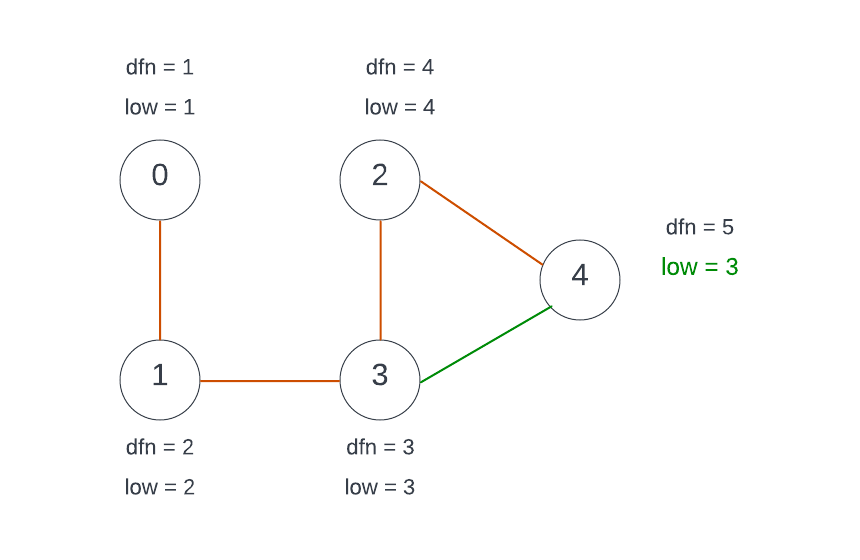Finding the articulation point
Now we check for every vertex v we need to compare the low[] value of a child node u with the depth first number of v, low[u] >= dfn[v] or low[child_node_of_vertex] >= dfn[vertex]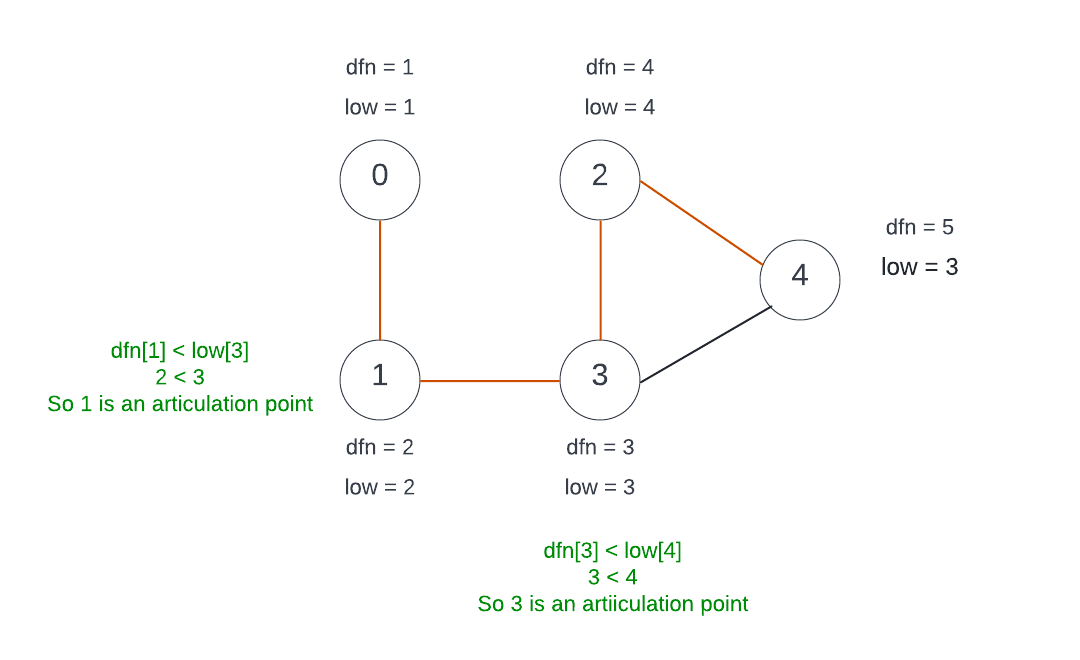## Applications of biconnected graph.

1. Articulation points could be more vulnerable and the failure of the articulation point vertex could lead to loss of function not just to the vertex but also between other vertices.
2. Biconnected graphs exhibit the property of redundancy and hence are highly useful in computer networks.
3. They could be used in situations where redundancy is ensured and single points of failure are undesirable.#### Sai Siri Chandana Namala

Graduate student of Computer Science at JNTUH College Of Engineering